# Optimal Control to The Rescue! Saving Us From Covid-19!

Last week, I mentioned that even with all of our social distancing efforts, here in the great state of Utah, we would still overwhelm the medical system. It was kind of a bleak grim reminder that we are dealing with the specter of impending death. Fortunately, COVID-19 is no black plague. Deadly, sure. Incurable, at the moment. But we can fight back with the limited tools that we have available to us. We can use optimal control theory to save the day.

So in this post, I hope that I will find a way to strike a cheerier note. I think that I can. A few of my loyal readers may have noticed very few graphs and charts. I did that on purpose last week because I don’t want to be irresponsible with data that is constantly in flux, that is probably inaccurate, and really scarier looking. This week, I am a little less concerned about my analysis. The truth of my analysis, is that it is highly speculative, takes a lot of assumptions which may or may not be good. This is just a demonstration of method, and hopefully shine a glimmer of hope for all of us out there. Please, for the love of all that is holy, do not interpret what I am doing here as gospel truth. It is not!

So let’s be upfront about the assumptions that I am making.

Assumption 1: It takes 14 days to recover from COVID-19.

Assumption 2: The basic reproduction number of the novel coronavirus, R0, is exactly 2.5.

These first two assumptions allow me to back out an infection rate for the disease. These assumptions shouldn’t be too controversial, but if you really must argue about them, that is what comments are for. The next set of assumptions are going to be a little bit more edgy.

Assumption 3: Social Distancing is not always perfectly adhered to. In fact, it is adhered to only 2% of the time.

Assumption 4: We also suck at finding people and quarantining them effectively. We only quarantine 1% of all cases effectively.

Assumption 5: Covid-19 will kill 2% of the people that get infected with it uniformly, which we just know isn’t true. And it will hospitalize 20% of the people that get infected. I’ve also tried to account for the fact that increasing the number of sick people will increase the number of dead by squaring the “death” terms.

Assumption 6: We care about people dying from COVID-19 100 times more than social distancing, and quarantining people.

Assumption 7: Social Distancing and Quarantine have real costs associated with them. And as mentioned people dying has a cost associated with it. These costs all grow quadraticly with the number of people in each group at a time. In other words if society consists of 6 people. 1 is social distancing the cost will be 1. Another 2 are quarantined, the cost for that group is 4. And 3 people died, that would cost 100X9=900.

Assumption 8: The disease will follow a basic SIR model, but one that I have modified to include the effects of social distancing, quarantines, and deaths. People can come in and out of social distancing, but not out of quarantines.

## Optimal Control

So given all of these assumptions we have everything that we need to build an optimal control model of COVID-19. So you might be asking yourself, what is optimal control? That is easy. It is simply a fairly complicated set of mathematical rules that optimizes a function subject to constraints that happen to be differential equations. That really is the best I can do for people that aren’t into this sort of stuff, sorry.

Anyway, rocket scientists use optimal control to figure out the best trajectories for space craft. Economists like me, use it to well cost minimize, or profit maximize, when there are time components involved. A good example would be finding an optimal production schedule, or the optimal way to extract oil. The trick with optimal control is that any decision that you make today will affect all of the decisions that you can make in the future. So you have to take into account the fact that even if something is good today, it might not be the best solution long-term.

My thought process here is that in the news I have been hearing about the economic costs around social distancing. Is this cure, worse than the disease economically speaking. Should we just let people die to keep people employed? Well, this framework will help us answer that exact question! That’s what a doctorate in economics buys you. So we can argue about the assumptions that I made, but not about the results with this model.

## The Math

Some quick notes on notation:

M represents the number of people that died. Q is the number of quarantined people. D is the number of people social distancing. S is the number of susceptible people. I is the number of infected people. R is the number of recovered people. $\beta$ is the infection rate. $\gamma$ is the recovery rate. $\delta$ is the mortality rate. $\epsilon$ is the coefficient determining the effectiveness of quarantines. $\eta$ is the coefficient determining the effectiveness of social distancing.

So the model looks something like this:

$\min_{x,y,z} \int_0^T 100M^2+Q^2+D^2 dt\\s.t.\\ \dot{S}=-\beta SI-\eta (xS-yD)\\ \dot{I}=\beta SI-\gamma I-\epsilon zI-\delta I^2\\ \dot{R}=\gamma (I+Q)\\ \dot{D}= \eta (xS-yD)\\ \dot{Q}=\epsilon zI-\gamma Q – \delta Q^2\\ \dot{M}=\delta (I^2+Q^2)$

If you go about trying to solve this particular math problem, after you find your first-order conditions you will have 15 equations, 12 of which are differential equations, and 15 unknowns to solve for. I started to solve this by hand, and then I realized that there was no way that I would find the analytical solution before the pandemic ended. So in a super lazy fashion, I turned to solving this problem numerically. It took about an hour to code it up, and then another 3 minutes or so to solve it.

One complaint that I had is that it doesn’t discount the objective function. So I care equally about future time-periods, as I do right now. The economist in me shudders, but let’s go with that for now.

## Coding the Optimal Control Problem

So like I said, I decided to solve this problem numerically instead of solving it analytically. Had I been writing this up as a research paper, I would be forced to come up with an analytical solution. I would also be looking for the comparative dynamics, and showing the relationship between different variables and the final path taken. We won’t do too much of that in this post. For now, let’s just look at the numerical simulation of this system.

To do that, we really need a numerical solver. Yet again, python comes to the rescue. Although you could slap something together using scipy, numpy, and their family. There is a ready to go package called gekko. I suggest pip installing that.

import matplotlib.animation as animation
import numpy as np
from gekko import GEKKO
import matplotlib.pyplot as plt

So these are my simple imports to make this whole thing happen. Initially, I was thinking about building a plot that was animated, but ultimately decided against it for the purposes of this post. Next we need to define a gekko model.

m = GEKKO()
m.solver_options = ['max_iter 300']
years = 2
# The amount of time we will give to the process
m.time = np.linspace(0,years*365,years*365+1)

You may have noticed in this piece of code that I decided to run the simulation over the course of 2 years. You can of course adjust that to whatever timeframe that you want, but 2 years seemed like a generous amount of time to develop a vaccine, especially, since we have viable options that are starting clinical trials soon.

#Set Parameter Values
beta = m.Param(value = 0.175) #Infection Rate
gamma = m.Param(value = 0.07) #Recovery Rate
epsilon = m.Param(value = 0.01) #Quarantine Effectiveness Coefficient
eta = m.Param(value = 0.02) #Social Distancing Effectiveness Coefficient
delta = m.Param(value = 0.02) #Mortality Rate
print("Implied R0 =",0.175/0.07)

All that we are doing in this block of code is setting a bunch of constants for the model to use. In the next block of code we will set our control variables x, y, and z. x controls the amount of social distancing efforts that society is going through. y controls the effort to turn social distancing off within society. You would think that these would just be mirror images of each other, but we shall see what is optimal. Finally, z controls our quarantine efforts. All three variables are bound between 0 and 1.

#Control variables
x = m.Var(value=0,lb=0,ub=1)
y = m.Var(value=0,lb=0,ub=1)
z = m.Var(value=0,lb=0,ub=1)

Next we need to define initial conditions. This is important because we will be dealing with differential equations, we need to know how to kick the system of equations off.

#State Variable Initial Conditions
S = m.SV(value=1)
I = m.SV(value=1000/3.16e6)
R = m.SV(value=0)
D = m.SV(value=0)
Q = m.SV(value=0)
M = m.SV(value=0)

The one thing that I will mention in terms of these initial conditions is that I am making an assumption about the total population size, and the total number of people that we are starting out with infected. No real rhyme or reason here, but I started out with 1000 people, and Utah’s population size of 3.16 million people. I’ve also normalized everything to work in percentages of the population. Now all that is left to do is to define how the system will evolve over time.

#Defining the State Space Model
m.Equation(S.dt() == -beta*S*I-eta*(x*S-y*D))
m.Equation(I.dt() == beta*S*I-gamma*I-epsilon*z*I-delta(I**2))
m.Equation(R.dt() == gamma*(I+Q))
m.Equation(D.dt() == eta*(x*S-y*D))
m.Equation(Q.dt() == epsilon*z*I-gamma*Q-delta*(Q**2))
m.Equation(M.dt() == delta*((Q**2)+(I**2)))

With that done, we just need to set an objective function, and solve the system. IMODE = 6, just tells gekko that we are doing an optimal control problem.

m.Obj(100*(M**2)+(D**2)+(Q**2))
m.options.IMODE = 6
m.solve()

And that’s it. You run that last block of code we’ll get a numerical solution to this optimal control problem. Pretty slick right?! The only thing left to do after the solver finishes its job is to get an idea of what the results look like by plotting the results.

plt.plot(m.time,np.array(I.value)+np.array(Q.value))
plt.title("Infections Over Time")
plt.ylabel("Proportion of Population\nInfected")
plt.xlabel("Days Into Outbreak")
plt.show()
plt.plot(m.time,np.array(S.value)+np.array(D.value))
plt.title("Suceptible Over Time")
plt.ylabel("Proportion of Population\nSuceptible")
plt.xlabel("Days Into Outbreak")
plt.show()
plt.plot(m.time,R.value)
plt.title("Recovered Over Time")
plt.ylabel("Proportion of Population\nRecovered")
plt.xlabel("Days Into Outbreak")
plt.show()
plt.plot(m.time,D.value)
plt.title("Social Distancing Over Time")
plt.ylabel("Proportion of Population\nSocial Distancing")
plt.xlabel("Days Into Outbreak")
plt.show()
plt.plot(m.time,Q.value)
plt.title("Quarantines Over Time")
plt.ylabel("Proportion of Population\nQuarantined")
plt.xlabel("Days Into Outbreak")
plt.show()
plt.plot(m.time,M.value)
plt.title("Deaths Over Time")
plt.ylabel("Proportion of Population\nDead")
plt.xlabel("Days Into Outbreak")
plt.show()
plt.plot(m.time,x.value,color='blue',label='Add')
plt.plot(m.time,y.value,color='red',label='Subtract')
plt.plot(m.time,z.value,color='green',label='Quarantine')
plt.legend()
plt.title("Controls Over Time")
plt.ylabel("Value of Controls")
plt.xlabel("Days Into Outbreak")
plt.show()

## The Results

Whew! That was a lot of plotting. I won’t show you what every graph looks like since that we be information overload. However, I will show you two graphs, the number of infected people over time, and the chart that shows how we should react to the epidemic, the controls, over time.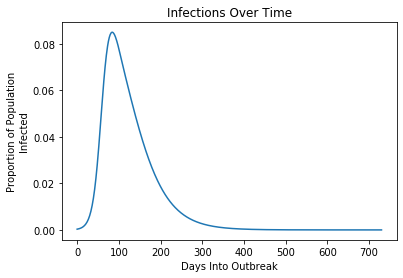Under optimal social distancing and optimal quarantines infections peak around day 84, with 8.5% of the population being sick at the same time.

It turns out that the peak of the outbreak happens when there is about 8.5% of the population infected. This of course means that the hospital system is still going to be overwhelmed. Again, going back to my assumptions a fifth of that 8.5% (or what would be called 1.7% of the population by normal people) will need to be hopitalized at the same time. For Utah that means about 53,600 people. We only have about 5000 beds, as I estimated last week. 66% of the population gets infected, and 25,000 people die in Utah. This optimal strategy obviously isn’t super optimal, but we’ll get back to fixing that in just a moment. We will add another constraint to the optimization process, but let’s take a look at those controls one more time.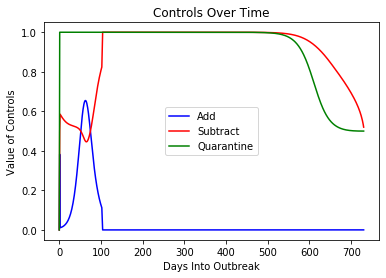Interestingly, some level of telling people to break the social distancing efforts appears to be optimal.

Here’s the thing that I find fascinating, there is no substitute for a good quarantine. The other thing that is optimal is that social distancing efforts start slowly, and ramp up until we reach the peak of the pandemic, and then drop off. Telling people to get out of the house and do their thing comes starts off at about 60% dips during the peak of the crisis, and then jumps all the way up to give an all clear once the pandemic abates naturally. These “all clear” messages are ever present in this optimal strategy. It is also consistent with what we are seeing POTUS doing. He’s saying things might be able to get back to normal by Easter. No one believes that but that would be true in this optimal strategy as well. Trump may have stumbled into this optimal strategy, where social distancing and quarantines are costly.

As mentioned this may be optimal, but it places a huge burden on the medical community and around 0.7% of the population dies as a result. Not the kind of solution that we are looking for.

So my question is, what if we add a constraint that says we aren’t allowed to let more hospitalization occur than our capacity? Is that even feasible with the dynamics of this disease?

## Adding The Most Important Constraint

So we can do this by adding one more equation to the model. This is really easy, we just have to add a single line of code. Just add the following before the call to m.solve().

#Do Not Overwhelm Hospital Constraint
m.Equation(0.2*(I+Q)*3.16e6 <= 5000) 

Adding this constraint turns out to be feasible! We can find a solution to the system!

This is what the infections overtime look like: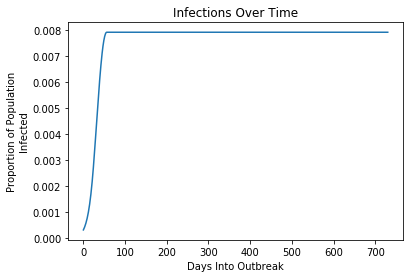With the “don’t overwhelm the hospitals” constraint in place infections never break 1%!

So in this scenario we end up ultimately having a near constant infection rate. We don’t spread out the curve, we completely stop it! You may be wondering how unpleasant will social distancing be in this world we’ve designed. And I have to say, it doesn’t look that bad. We’ll be doing some form of it for years to come, but things will slowly and steadily get better and better.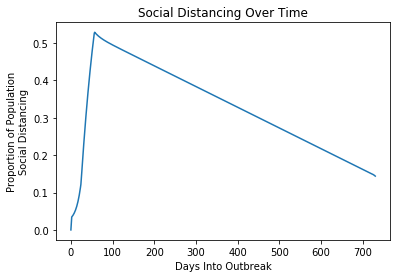We get up over 50% of the population practices social distancing quickly, and then immediately start backing that down slowly.

It looks like we are in for aggressive social distancing for approximately 3 months under this scenario. And then we start backing that down. It doesn’t fully go away, but normalcy should start coming back around a little bit after that. Restaurants and bars reopen, but they are less crowded. Sports resume, but only for the cameramen. You get the idea.

So how do we achieve this outcome, it seems magical the way that these numbers stay soooo low.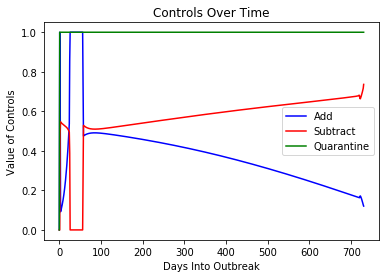Early days of the pandemic, it is okay to talk about staying out and doing things, but almost overnight, we change our tune.

It appears that this strategy of social distancing is designed to give health departments time to do tracing and get people quarantined. Once we have a handle on the situation using quarantines, life slowly gets back to normal. One thing that I want to note is that you turn the dial all the way to 100% on social distancing for about a month, simultaneously you turn the dial on telling people it is okay to go out all the way to 0 during this time period. It is unambiguously bad to confuse people during this time period.

Once again some messaging at the beginning of the outbreak to go about your business and not panic too much about the outbreak is optimal. It isn’t until you are about 30 days into the pandemic that the tone needs to suddenly and dramatically shift. At that point you get another 30 days of do nothing, stay home. And then you slowly start to send out the message that things are okay more and more. Again at the beginning of this thing, it appears that Trump is sending the right messages. He needs to change his tone pretty quickly though. He will quickly walk right out of the optimal strategy. So he doesn’t quite get passing grades yet, but he does get me to cut him a break for now.

So that is an optimal control for this pandemic. Let’s see what our pandemic looks like for Utah. After 2 years, we “peak” at 0.8% of the population has been infected, that feels like black magic. We never exceed our 5000 bed capacity at the hospitals. Around 39% of the population gets infected with COVID-19, but only 2154 people die by the end of 2 years.

Personally, I think that this policy regime seems to look a lot like what went on in China. They did really restrictive social distancing for about a month or two, and then once they had a handle on the situation, they switched to a tracing and quarantine strategy, which is what this says you should do if you do not want to overwhelm your medical system and social distancing is expensive. Right down to them down-playing the epidemic in the beginning of the crisis. Optimal control really helped us find out what we should do.

Remember, we can talk about the assumptions that I made, but these results are essentially bulletproof insofar as my assumptions are bulletproof. So if you accept my assumptions, then you can’t argue. Of course, that is the name of the game with these types of models, making the right assumptions. If you want to change the assumptions, and play around with the model, then I want to hear about what you did, it sounds cool. Did you mess around and get completely different results than me? Let me know in the comments. I would love to see what assumptions you made and what your optimal control problem tells you what we should do.

The big thing that I want to emphasize is that these models tell us a story. The story is that it is completely feasible to turn the corner on this novel coronavirus. That being said, we do need to take our medicine, no matter how bad it tastes. If we do that, we can not only flatten the curve, we can control the curve. If we follow this program, we can avoid overwhelming the medical system, minimize the number of deaths, and get things to some semblance of normal.

Published

## 3 comments

1.Erik Hannesson says:

Nice article. My *only* issue with it is that, strictly speaking, optimal control theory optimizes functionals, not functions 😉 but I think you made the better choice avoiding trying to explain the difference haha

1.Ryan Barnes says:

Ha! I put this together so fast I didn’t realize that I made that mistake, even though I knew better. However, now that you bring it up, it does seem like I’d be splitting hairs for a lay audience. I think that you’re right and I won’t fix that particular mistake.